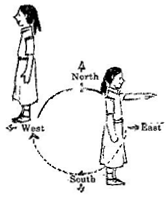1. /
2. CBSE
3. /
4. Class 06
5. /
6. Mathematics
7. /
8. NCERT Solutions for Class...

# NCERT Solutions for Class 6 Maths Exercise 5.2

NCERT solutions for Maths Understanding elementary## NCERT Solutions for Class 6 Maths Understanding elementary

###### Question 1.What fraction of a clockwise revolution does the hour hand of a clock turn through, when it goes from

(a) 3 to 9, (b) 4 to 7, (c) 7 to 10, (d) 12 to 9, (e) 1 to 10, (f) 6 to 3

(a) {tex}\frac{1}{2}{/tex} or two right angles

(b) {tex}\frac{1}{4}{/tex} or oneright angle

(c) {tex}\frac{1}{4}{/tex} or one right angle

(d) {tex}\frac{3}{4}{/tex} or three right angles.

(e) {tex}\frac{3}{4}{/tex} or three right angles.

(f) {tex}\frac{3}{4}{/tex} or three right angles.

###### Question 2.Where will the hand of a clock stop if it:

(a)starts at 12 and make {tex}\frac{1}{2}{/tex} of a revolution, clockwise?

(b)starts at 2 and makes {tex}\frac{1}{2}{/tex} of a revolution, clockwise?

(c)starts at 5 and makes {tex}\frac{1}{4}{/tex} of a revolution, clockwise?

(d)starts at 5 and makes {tex}\frac{3}{4}{/tex} of a revolution, clockwise?

Answer: (a) At 6, (b) At 8, (c) At 8, (d) At 2

NCERT Solutions for Class 6 Maths Exercise 5.2

###### Question 3.Which direction will you face if you start facing:(a)East and make {tex}\frac{1}{2}{/tex} of a revolution clockwise?

(b)East and make 1{tex}\frac{1}{2}{/tex} of a revolution clockwise?

(c)West and makes {tex}\frac{3}{4}{/tex} of a revolution, clockwise?

(d)South and make one full revolution?

(Should we specify clockwise or anti-clockwise for this last question? Why not?)

Answer: (a) West, (b) West , (c) North, (d) South

(For answer (d), it is immaterial whether we turn clockwise or anticlockwise, because one full revolution will bring us back to the original position)

###### Question 4.What part of a revolution have you turned through if you stand facing:

(a)East and turn clockwise to face north?

(b)South and turn clockwise to fase east?

(c)West and turn clockwise to face east?

(a) {tex}\frac{3}{4}{/tex}

(b) {tex}\frac{3}{4}{/tex}

(c) {tex}\frac{1}{2}{/tex}

NCERT Solutions for Class 6 Maths Exercise 5.2

###### Question 5.Find the number of right angles turned through by the hour hand of a clock when it goes from:

(a) 3 to 6, (b) 2 to 8, (c) 5 to 11, (d) 10 to 1, (e) 12 to 9, (f) 12 to 6

(a) One right angle

(b) Two right angles

(c) Two right angles

(d) One right angle

(e) Three right angles

(f) Two right angles

NCERT Solutions for Class 6 Maths Exercise 5.2

###### Question 6.How many right angles do you make if you start facing:

(a)South and turn clockwise to west?

(b)North and turn anti-clockwise to east?

(c)West and turn to west?

(d)South and turn to north?

(a) One right angle

(b) Three right angles

(c) Four right angles

(d) Two right angles

NCERT Solutions for Class 6 Maths Exercise 5.2

###### Question 7. Where will the hour hand of a clock stop if it starts:

(a)from 6 and turns through 1 right angle?

(b)from 8 and turns through 2 right angles?

(c)from 10 and turns through 3 right angles?

(d)from 7 and turns through 2 straight angles?

Answer: (a) At 9, (b) At 2, (c) At 7, (d) At 7

## NCERT Solutions for Class 6 Maths Exercise 5.2

NCERT Solutions Class 6 Maths PDF (Download) Free from myCBSEguide app and myCBSEguide website. Ncert solution class 6 Maths includes text book solutions from Class 6 Maths Book . NCERT Solutions for CBSE Class 6 Maths have total 14 chapters. 6 Maths NCERT Solutions in PDF for free Download on our website. Ncert Maths class 6 solutions PDF and Maths ncert class 6 PDF solutions with latest modifications and as per the latest CBSE syllabus are only available in myCBSEguide.

## CBSE app for Students

To download NCERT Solutions for Class 6 Maths, Social Science Computer Science, Home Science, Hindi English, Maths Science do check myCBSEguide app or website. myCBSEguide provides sample papers with solution, test papers for chapter-wise practice, NCERT solutions, NCERT Exemplar solutions, quick revision notes for ready reference, CBSE guess papers and CBSE important question papers. Sample Paper all are made available through the best app for CBSE students and myCBSEguide website.Test Generator

Create question paper PDF and online tests with your own name & logo in minutes.myCBSEguide

Question Bank, Mock Tests, Exam Papers, NCERT Solutions, Sample Papers, Notes